Get inspired by the success stories of our students in IIT JAM MS, ISI  MStat, CMI MSc DS.  Learn More

# ISI MStat PSB 2013 Problem 9 | Envelope Collector's ExpenditureThis is a very simple and beautiful sample problem from ISI MStat PSB 2013 Problem 9. It is mainly based on geometric distribution and its expectation . Try it!

## Problem- ISI MStat PSB 2013 Problem 9

Envelopes are on sale for Rs. 30 each. Each envelope contains exactly one coupon, which can be one of the one of four types with equal probability. Suppose you keep on buying envelopes and stop when you collect all the four type of coupons. What will be your expenditure ?

### Prerequisites

Geometric Distribution

Expectation of geometric distribution

Basic counting

## Solution :

This problem seems quite simple and it is simple, often one may argue that we can take a single random variable, which denotes the number of trials till the fourth success (or is it third !!), and calculate its expectation. But I differ here becaue I find its lot easier to work with sum of geometric random variables than a negative binomial. ( negative binomial is actually sum of finite geometrics !!)

So, here what we will do, is define 4 random variables, as: # trials to get a type of coupon that is different from the all the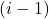types of coupons drawn earlier.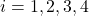.

Now since each type of coupon has an equal probability to come, that is probability of success is, here a common mistakes people commit is assuming all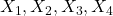are i.i.d Geometric(), and this turns out to be a disaster !! So, be aware and observe keenly, that at the first draw, any of the four types will come, with probability 1, and there after we just need the rest of the 3 types to appear at least once. So, here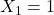always and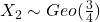( becuase since in the first trial, surely any of the four types will come, our success would be getting any of the 3 types of envelopes from the all types making the success probability) similarly ,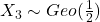and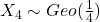.

Now for the expectated expenditure at the given rate of Rs. 30 per envelope, expected expenditure is Rs.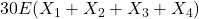Now, we know that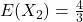,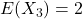and(why??)

So,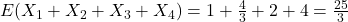.

So, our required expectation is Rs. 250. Hence we are done !

## Food For Thought

Suppose among the envelopes you collected each envelope has an unique 5 digit number, you picked an envelope randomly and what is the chance of the number that is going to show up is has its digits in a non-decreasing order ?

How does the chances may vary if you find a k digit number on the envelope ? think over it !!

## Subscribe to Cheenta at Youtube

This is a very simple and beautiful sample problem from ISI MStat PSB 2013 Problem 9. It is mainly based on geometric distribution and its expectation . Try it!

## Problem- ISI MStat PSB 2013 Problem 9

Envelopes are on sale for Rs. 30 each. Each envelope contains exactly one coupon, which can be one of the one of four types with equal probability. Suppose you keep on buying envelopes and stop when you collect all the four type of coupons. What will be your expenditure ?

### Prerequisites

Geometric Distribution

Expectation of geometric distribution

Basic counting

## Solution :

This problem seems quite simple and it is simple, often one may argue that we can take a single random variable, which denotes the number of trials till the fourth success (or is it third !!), and calculate its expectation. But I differ here becaue I find its lot easier to work with sum of geometric random variables than a negative binomial. ( negative binomial is actually sum of finite geometrics !!)

So, here what we will do, is define 4 random variables, as: # trials to get a type of coupon that is different from the all thetypes of coupons drawn earlier..

Now since each type of coupon has an equal probability to come, that is probability of success is, here a common mistakes people commit is assuming allare i.i.d Geometric(), and this turns out to be a disaster !! So, be aware and observe keenly, that at the first draw, any of the four types will come, with probability 1, and there after we just need the rest of the 3 types to appear at least once. So, herealways and( becuase since in the first trial, surely any of the four types will come, our success would be getting any of the 3 types of envelopes from the all types making the success probability) similarly ,and.

Now for the expectated expenditure at the given rate of Rs. 30 per envelope, expected expenditure is Rs.Now, we know that,and(why??)

So,.

So, our required expectation is Rs. 250. Hence we are done !

## Food For Thought

Suppose among the envelopes you collected each envelope has an unique 5 digit number, you picked an envelope randomly and what is the chance of the number that is going to show up is has its digits in a non-decreasing order ?

How does the chances may vary if you find a k digit number on the envelope ? think over it !!

## Subscribe to Cheenta at Youtube

This site uses Akismet to reduce spam. Learn how your comment data is processed.

### Knowledge Partner